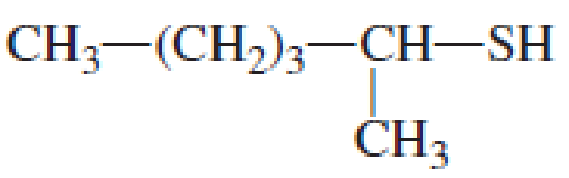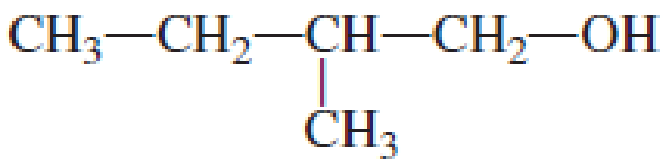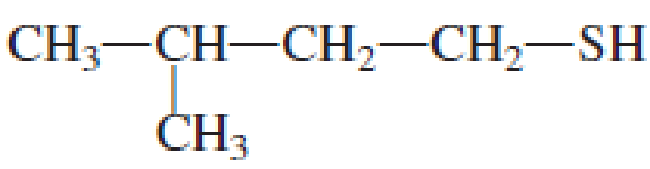Chapter 3, Problem 3.140EP### Organic And Biological Chemistry

7th Edition
STOKER + 1 other
ISBN: 9781305081079

#### Solutions

Chapter
Section### Organic And Biological Chemistry

7th Edition
STOKER + 1 other
ISBN: 9781305081079
Textbook Problem

# Assign an IUPAC name to each of the following compounds.(a)

Interpretation Introduction

Interpretation:

IUPAC name for the given compound has to be assigned.

Concept Introduction:

IUPAC rules for naming thioalcohols that contain single thiol group:

• • Longest carbon chain has to be identified that contains thiol group also.  The chain name is obtained by adding the suffix “-thiol”.  If the compound contains an unsaturated bond, then the respective name has to be changed with regard to alkane.
• • The numbering has to be given so that the thiol group gets the least numbering.
• • Name and location of any other substituent present in the chain has to be identified.
• • If in a ring the thiol group is present, then that carbon is numbered 1 and the numbering then proceeds counterclockwise or clockwise in a way that substituents present if any gets the least numbering.

IUPAC rules for naming thioalcohols that contain more than one thiol group:

• • The same rules said above is followed but the prefix di-, tri-, tetra etc is added corresponding to the number of thiol groups that is present.
Explanation

Given structure of compound is shown below,

First step is to identify the longest continuous carbon chain.  In the given structure, it is found that the longest carbon chain is six carbon chain.  Hence, the parent alkane is hexane.

(b)

Interpretation Introduction

Interpretation:

IUPAC name for the given compound has to be assigned.

Concept Introduction:

IUPAC rules for naming alcohols that contain single hydroxyl group:

• • Longest carbon chain has to be identified that contains hydroxyl group also.  The chain name is obtained by replacing the letter “-e” in alkane with “-ol”.  If the compound contains a unsaturated bond, then the respective name has to be changed with regard to alkane.
• • The numbering has to be given so that the hydroxyl group gets the least numbering.
• • Name and location of any other substituent present in the chain has to be identified.
• • If in a ring the hydroxyl group is present, then that carbon is numbered 1 and the numbering then proceeds counterclockwise or clockwise in a way that substituents present if any gets the least numbering.
• • Hydroxyl group as a substituent in a molecule is named as hydroxy group rather than hydroxyl group.
• • If the compound contains bulky groups on same side of the double bond, then it is a cis isomer and if the bulkyl groups are present on opposite side of the double bond, then it is a trans isomer.
• • In case of cycloalkane compounds, if the substitutions are present on same side of the ring of carbon atoms, it is a cis isomer.  If the substitutions are present above and below the ring, then it is a trans isomer.

IUPAC rules for naming alcohols that contain more than one hydroxyl group:

• • The same rules said above is followed but the prefix di-, tri-, tetra etc is added corresponding to the number of hydroxyl groups that is present.

(c)

Interpretation Introduction

Interpretation:

IUPAC name for the given compound has to be assigned.

Concept Introduction:

IUPAC rules for naming alcohols that contain single hydroxyl group:

• • Longest carbon chain has to be identified that contains hydroxyl group also.  The chain name is obtained by replacing the letter “-e” in alkane with “-ol”.  If the compound contains a unsaturated bond, then the respective name has to be changed with regard to alkane.
• • The numbering has to be given so that the hydroxyl group gets the least numbering.
• • Name and location of any other substituent present in the chain has to be identified.
• • If in a ring the hydroxyl group is present, then that carbon is numbered 1 and the numbering then proceeds counterclockwise or clockwise in a way that substituents present if any gets the least numbering.
• • Hydroxyl group as a substituent in a molecule is named as hydroxy group rather than hydroxyl group.
• • If the compound contains bulky groups on same side of the double bond, then it is a cis isomer and if the bulkyl groups are present on opposite side of the double bond, then it is a trans isomer.
• • In case of cycloalkane compounds, if the substitutions are present on same side of the ring of carbon atoms, it is a cis isomer.  If the substitutions are present above and below the ring, then it is a trans isomer.

IUPAC rules for naming alcohols that contain more than one hydroxyl group:

• • The same rules said above is followed but the prefix di-, tri-, tetra etc is added corresponding to the number of hydroxyl groups that is present.

(d)

Interpretation Introduction

Interpretation:

IUPAC name for the given compound has to be assigned.

Concept Introduction:

IUPAC rules for naming thioalcohols that contain single thiol group:

• • Longest carbon chain has to be identified that contains thiol group also.  The chain name is obtained by adding the suffix “-thiol”.  If the compound contains an unsaturated bond, then the respective name has to be changed with regard to alkane.
• • The numbering has to be given so that the thiol group gets the least numbering.
• • Name and location of any other substituent present in the chain has to be identified.
• • If in a ring the thiol group is present, then that carbon is numbered 1 and the numbering then proceeds counterclockwise or clockwise in a way that substituents present if any gets the least numbering.

IUPAC rules for naming thioalcohols that contain more than one thiol group:

• • The same rules said above is followed but the prefix di-, tri-, tetra etc is added corresponding to the number of thiol groups that is present.

### Still sussing out bartleby?

Check out a sample textbook solution.

See a sample solution

#### The Solution to Your Study Problems

Bartleby provides explanations to thousands of textbook problems written by our experts, many with advanced degrees!

Get Started